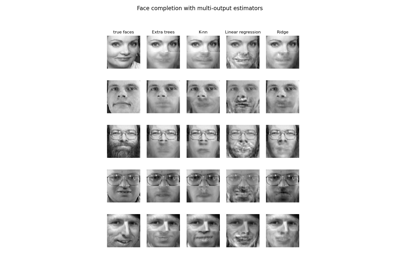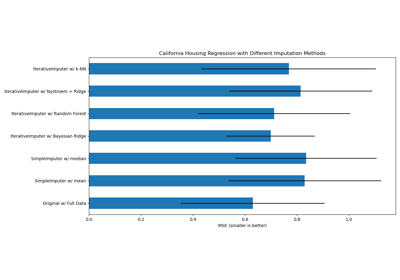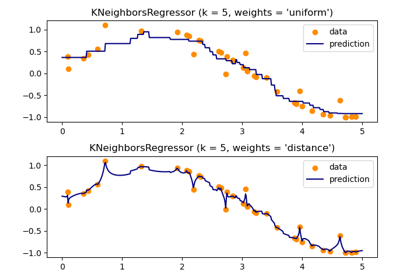# sklearn.neighbors.KNeighborsRegressor¶

class sklearn.neighbors.KNeighborsRegressor(n_neighbors=5, *, weights='uniform', algorithm='auto', leaf_size=30, p=2, metric='minkowski', metric_params=None, n_jobs=None, **kwargs)[source]

Regression based on k-nearest neighbors.

The target is predicted by local interpolation of the targets associated of the nearest neighbors in the training set.

Read more in the User Guide.

New in version 0.9.

Parameters
n_neighborsint, default=5

Number of neighbors to use by default for kneighbors queries.

weights{‘uniform’, ‘distance’} or callable, default=’uniform’

weight function used in prediction. Possible values:

• ‘uniform’ : uniform weights. All points in each neighborhood are weighted equally.

• ‘distance’ : weight points by the inverse of their distance. in this case, closer neighbors of a query point will have a greater influence than neighbors which are further away.

• [callable] : a user-defined function which accepts an array of distances, and returns an array of the same shape containing the weights.

Uniform weights are used by default.

algorithm{‘auto’, ‘ball_tree’, ‘kd_tree’, ‘brute’}, default=’auto’

Algorithm used to compute the nearest neighbors:

Note: fitting on sparse input will override the setting of this parameter, using brute force.

leaf_sizeint, default=30

Leaf size passed to BallTree or KDTree. This can affect the speed of the construction and query, as well as the memory required to store the tree. The optimal value depends on the nature of the problem.

pint, default=2

Power parameter for the Minkowski metric. When p = 1, this is equivalent to using manhattan_distance (l1), and euclidean_distance (l2) for p = 2. For arbitrary p, minkowski_distance (l_p) is used.

metricstr or callable, default=’minkowski’

the distance metric to use for the tree. The default metric is minkowski, and with p=2 is equivalent to the standard Euclidean metric. See the documentation of DistanceMetric for a list of available metrics. If metric is “precomputed”, X is assumed to be a distance matrix and must be square during fit. X may be a sparse graph, in which case only “nonzero” elements may be considered neighbors.

metric_paramsdict, default=None

Additional keyword arguments for the metric function.

n_jobsint, default=None

The number of parallel jobs to run for neighbors search. None means 1 unless in a joblib.parallel_backend context. -1 means using all processors. See Glossary for more details. Doesn’t affect fit method.

Attributes
effective_metric_str or callable

The distance metric to use. It will be same as the metric parameter or a synonym of it, e.g. ‘euclidean’ if the metric parameter set to ‘minkowski’ and p parameter set to 2.

effective_metric_params_dict

Additional keyword arguments for the metric function. For most metrics will be same with metric_params parameter, but may also contain the p parameter value if the effective_metric_ attribute is set to ‘minkowski’.

n_samples_fit_int

Number of samples in the fitted data.

Notes

See Nearest Neighbors in the online documentation for a discussion of the choice of algorithm and leaf_size.

Warning

Regarding the Nearest Neighbors algorithms, if it is found that two neighbors, neighbor k+1 and k, have identical distances but different labels, the results will depend on the ordering of the training data.

https://en.wikipedia.org/wiki/K-nearest_neighbor_algorithm

Examples

>>> X = [, , , ]
>>> y = [0, 0, 1, 1]
>>> from sklearn.neighbors import KNeighborsRegressor
>>> neigh = KNeighborsRegressor(n_neighbors=2)
>>> neigh.fit(X, y)
KNeighborsRegressor(...)
>>> print(neigh.predict([[1.5]]))
[0.5]


Methods

 fit(X, y) Fit the k-nearest neighbors regressor from the training dataset. get_params([deep]) Get parameters for this estimator. kneighbors([X, n_neighbors, return_distance]) Finds the K-neighbors of a point. kneighbors_graph([X, n_neighbors, mode]) Computes the (weighted) graph of k-Neighbors for points in X Predict the target for the provided data score(X, y[, sample_weight]) Return the coefficient of determination R^2 of the prediction. set_params(**params) Set the parameters of this estimator.
fit(X, y)[source]

Fit the k-nearest neighbors regressor from the training dataset.

Parameters
X{array-like, sparse matrix} of shape (n_samples, n_features) or (n_samples, n_samples) if metric=’precomputed’

Training data.

y{array-like, sparse matrix} of shape (n_samples,) or (n_samples, n_outputs)

Target values.

Returns
selfKNeighborsRegressor

The fitted k-nearest neighbors regressor.

get_params(deep=True)[source]

Get parameters for this estimator.

Parameters
deepbool, default=True

If True, will return the parameters for this estimator and contained subobjects that are estimators.

Returns
paramsmapping of string to any

Parameter names mapped to their values.

kneighbors(X=None, n_neighbors=None, return_distance=True)[source]

Finds the K-neighbors of a point. Returns indices of and distances to the neighbors of each point.

Parameters
Xarray-like, shape (n_queries, n_features), or (n_queries, n_indexed) if metric == ‘precomputed’

The query point or points. If not provided, neighbors of each indexed point are returned. In this case, the query point is not considered its own neighbor.

n_neighborsint

Number of neighbors to get (default is the value passed to the constructor).

return_distanceboolean, optional. Defaults to True.

If False, distances will not be returned

Returns
neigh_distarray, shape (n_queries, n_neighbors)

Array representing the lengths to points, only present if return_distance=True

neigh_indarray, shape (n_queries, n_neighbors)

Indices of the nearest points in the population matrix.

Examples

In the following example, we construct a NearestNeighbors class from an array representing our data set and ask who’s the closest point to [1,1,1]

>>> samples = [[0., 0., 0.], [0., .5, 0.], [1., 1., .5]]
>>> from sklearn.neighbors import NearestNeighbors
>>> neigh = NearestNeighbors(n_neighbors=1)
>>> neigh.fit(samples)
NearestNeighbors(n_neighbors=1)
>>> print(neigh.kneighbors([[1., 1., 1.]]))
(array([[0.5]]), array([]))


As you can see, it returns [[0.5]], and [], which means that the element is at distance 0.5 and is the third element of samples (indexes start at 0). You can also query for multiple points:

>>> X = [[0., 1., 0.], [1., 0., 1.]]
>>> neigh.kneighbors(X, return_distance=False)
array([,
]...)

kneighbors_graph(X=None, n_neighbors=None, mode='connectivity')[source]

Computes the (weighted) graph of k-Neighbors for points in X

Parameters
Xarray-like, shape (n_queries, n_features), or (n_queries, n_indexed) if metric == ‘precomputed’

The query point or points. If not provided, neighbors of each indexed point are returned. In this case, the query point is not considered its own neighbor.

n_neighborsint

Number of neighbors for each sample. (default is value passed to the constructor).

mode{‘connectivity’, ‘distance’}, optional

Type of returned matrix: ‘connectivity’ will return the connectivity matrix with ones and zeros, in ‘distance’ the edges are Euclidean distance between points.

Returns
Asparse graph in CSR format, shape = [n_queries, n_samples_fit]

n_samples_fit is the number of samples in the fitted data A[i, j] is assigned the weight of edge that connects i to j.

Examples

>>> X = [, , ]
>>> from sklearn.neighbors import NearestNeighbors
>>> neigh = NearestNeighbors(n_neighbors=2)
>>> neigh.fit(X)
NearestNeighbors(n_neighbors=2)
>>> A = neigh.kneighbors_graph(X)
>>> A.toarray()
array([[1., 0., 1.],
[0., 1., 1.],
[1., 0., 1.]])

predict(X)[source]

Predict the target for the provided data

Parameters
Xarray-like of shape (n_queries, n_features), or (n_queries, n_indexed) if metric == ‘precomputed’

Test samples.

Returns
yndarray of shape (n_queries,) or (n_queries, n_outputs), dtype=int

Target values.

score(X, y, sample_weight=None)[source]

Return the coefficient of determination R^2 of the prediction.

The coefficient R^2 is defined as (1 - u/v), where u is the residual sum of squares ((y_true - y_pred) ** 2).sum() and v is the total sum of squares ((y_true - y_true.mean()) ** 2).sum(). The best possible score is 1.0 and it can be negative (because the model can be arbitrarily worse). A constant model that always predicts the expected value of y, disregarding the input features, would get a R^2 score of 0.0.

Parameters
Xarray-like of shape (n_samples, n_features)

Test samples. For some estimators this may be a precomputed kernel matrix or a list of generic objects instead, shape = (n_samples, n_samples_fitted), where n_samples_fitted is the number of samples used in the fitting for the estimator.

yarray-like of shape (n_samples,) or (n_samples, n_outputs)

True values for X.

sample_weightarray-like of shape (n_samples,), default=None

Sample weights.

Returns
scorefloat

R^2 of self.predict(X) wrt. y.

Notes

The R2 score used when calling score on a regressor uses multioutput='uniform_average' from version 0.23 to keep consistent with default value of r2_score. This influences the score method of all the multioutput regressors (except for MultiOutputRegressor).

set_params(**params)[source]

Set the parameters of this estimator.

The method works on simple estimators as well as on nested objects (such as pipelines). The latter have parameters of the form <component>__<parameter> so that it’s possible to update each component of a nested object.

Parameters
**paramsdict

Estimator parameters.

Returns
selfobject

Estimator instance.

## Examples using sklearn.neighbors.KNeighborsRegressor¶